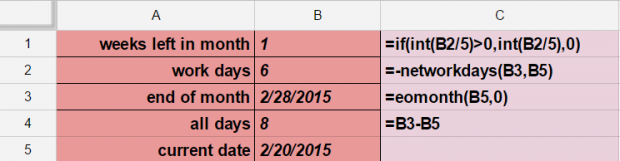# Google Sheets: How to Work with Dates

### Use Date Math with Google Sheets

This tutorial demonstrates how to use date math with the following computations:

• Count the number of work days between two days
• Count the total number of days between two dates
• Find the last day in the month
• Determine how many weeks are left in the month

Here is how to make each of these calculations and the formulas needed to do so.  Below the graphic, I explain the formulas.### Find the Number of Weeks Left in the Month

The function below uses the number of weekdays left in the month divided by five, since there are five days in the work week, to determine how many weeks are left in the month.  You could also take the number of days left in the month and divide by seven.  Note that it is necessary to use the int function to chop off the decimal point since, for example, it would not make much sense to say that there are 3.2 weeks left per month.

if(int(B2/5)>0,int(B2/5),0)

### Find the Number of Working Days between Two Dates

The networkdays functions find the number of workdays between two dates.  In order to exclude holidays, you would have to list them as the last argument in the forumla.

networkdays(B3,B5)

### Find the Last Day of the Month

The eomonth function shows the last day in the month.

eomonth(b5,0)

### Find the Number of Days between Two Dates

Since dates are numbers, you can just subtract one date from another.

B3-B5

RELATED ARTICLES